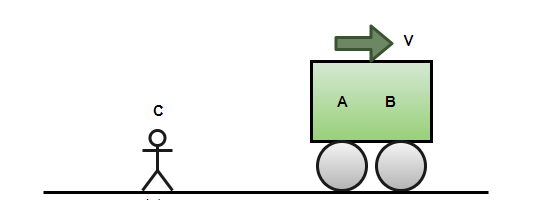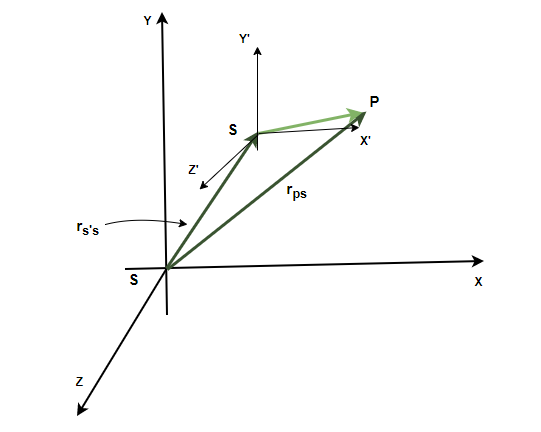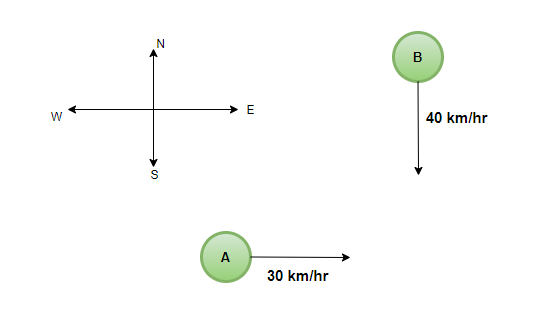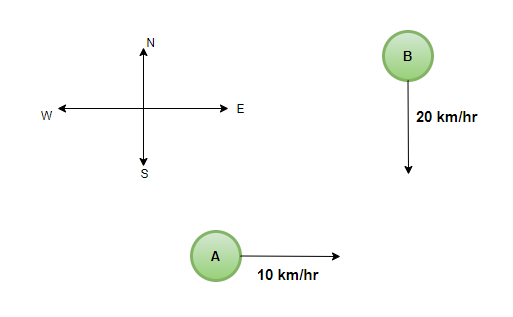Related Articles

# Relative Motion in Two Dimension

• Last Updated : 05 Aug, 2021

The motion of the bodies is not absolute or isolated. It is always described with respect to some reference. For example, the speed of a moving vehicle is measured with respect to the ground. The position is also measured with respect to a reference which is called the origin. A train moving has a velocity of 100 Km/h with respect to the ground, but if another train is moving at 150Km/h. The velocity of the first train will not be 100Km/h with respect to the person sitting on the second train. It is essential to study the relative motion of the objects. Let’s explore this concept in detail.

### Reference Frames

In physics, a frame of reference is an abstract coordinate system, and a set of physical reference points that uniquely fix the coordinate system help in standardizing the measurements with respect to that frame. Consider the figure below, the velocity of the cart with respect to the boy is non-zero, but the velocity of the cart with respect to the person sitting inside the car is zero.### Relative Motion in One Dimension

Relative Motion in one dimension is the building block for calculations related to relative motions in more than one dimension. Vectors can be decomposed into their components and then this rule can be used to calculate the relative velocity in different directions. As an example, consider a person sitting on a train moving at 10m/s towards the east. Assuming that the east direction is chosen as the positive direction and the earth as a reference frame.

The velocity of the train with respect to the ground frame,

vTE = 10 m/s

Now let’s say a person on the train starts getting up and starts moving in the direction opposite to the train. He is moving at 2m/s inside the train. This velocity is with respect to the reference of the train’s frame. The velocity of the person is,

vPT = -2 m/s

These two vectors can be added to find the velocity of the person with respect to the earth. This is called relative velocity.

vPE = vPT + vTE

### Relative Motion in Two Dimensions

These concepts can be extended to two-dimensional spaces also. Given in the figure below, consider a particle P and reference frames S and S’. The position of the frame S’ as measured in S is rS’S, the position of the particle P as measured with respect to the frame S’ is given by rPS’ and the position of the particle P with respect to the frame of reference S is given by rPS,Notice from the figure that,

rPS  = rPS’ + rS’S

These vectors give us the formula for relative velocities too, differentiating the above equation,Intuitively speaking, the velocity of a particle with respect to S is equal to the velocity of S’ with respect to S plus the velocity of the particle with respect to S.

Differentiating this equation again, the equation for the acceleration is given by,The acceleration of a particle with respect to S is equal to the acceleration of S’ with respect to S plus the acceleration of the particle with respect to S.

### Sample Problems

Question 1: A train is moving at a speed of 100 Km/h. The person sitting inside the train starts moving in the direction of the train at a speed of 10Km/h. Find the velocity of the person with respect to the ground.

Given:

velocity of the train with respect to the ground, vTG = 100Km/h

velocity of the person with respect to the train, vPT = 10Km/h

velocity of the person with respect to the ground vPG,

The equation mentioned above states,

vPG  = vPT + vTG

plugging the values into the above equation,

⇒ vPG  = 100 + 10

⇒ vPG  = 110

Question 2: A train is moving at a speed of 100 Km/h. The person sitting inside the train starts moving in the opposite direction of the train at a speed of 10Km/h. Find the velocity of the person with respect to the ground.

Given:

velocity of the train with respect to the ground, vTG = 100Km/h

velocity of the person with respect to the train, vPT = -10Km/h

velocity of the person with respect to the ground vPG,

The equation mentioned above states,

vPG  = vPT + vTG

plugging the values into the above equation,

⇒ vPG  = 100 – 10

⇒ vPG  = 90

Question 3: A vehicle is moving at a speed of 3i + 4j m/s. The person inside the car thinks the bird is flying at a velocity of 2i + 2j m/s. Find the speed of the bird with respect to the direct.

Given:

velocity of the vehicle with respect to the ground, vVG = 3i + 4j

velocity of the bird with respect to the vehicle, vBV = 2i + 2j

velocity of the person with respect to the ground vBG,

The equation mentioned above states,

vBG  = vBV + vVG

plugging the values into the above equation,

⇒ vPG  = 2i + 2j + 3i + 4j

⇒ vPG  = 5i + 6j

⇒  |vPG|  = √61 m/s

Question 4: Two particles A and B are moving with velocities 30Km/h and 40Km/h respectively towards an intersection as shown in the figure. Find the velocity of particle A with respect to the velocity of particle B.Given: vA = 30 Km/h and  vB = 40 Km/h.

Figure shows they are moving in perpendicular directions.

Velocity of A with respect to earth: vAE = 30i

Velocity of B with respect to earth: vBE = -40j

Using the equation studied above, velocity of A with respect to B: vAB

Assuming that the earth is the connecting frame of reference here,⇒⇒magnitude of this velocity is⇒⇒Question 5: Two particles A and B are moving with velocities 10Km/h and 20Km/h respectively towards an intersection as shown in the figure. Find the velocity of particle A with respect to the velocity of particle B.Given: vA = 10 Km/h and  vB = 20 Km/h.

Figure shows they are moving in perpendicular directions.

Velocity of A with respect to earth: vAE = 10i

Velocity of B with respect to earth: vBE = -20j

Using the equation studied above, velocity of A with respect to B: vAB

Assuming that the earth is the connecting frame of reference here,⇒⇒magnitude of this velocity is⇒⇒Attention reader! All those who say programming isn’t for kids, just haven’t met the right mentors yet. Join the  Demo Class for First Step to Coding Course, specifically designed for students of class 8 to 12.

The students will get to learn more about the world of programming in these free classes which will definitely help them in making a wise career choice in the future.

My Personal Notes arrow_drop_up